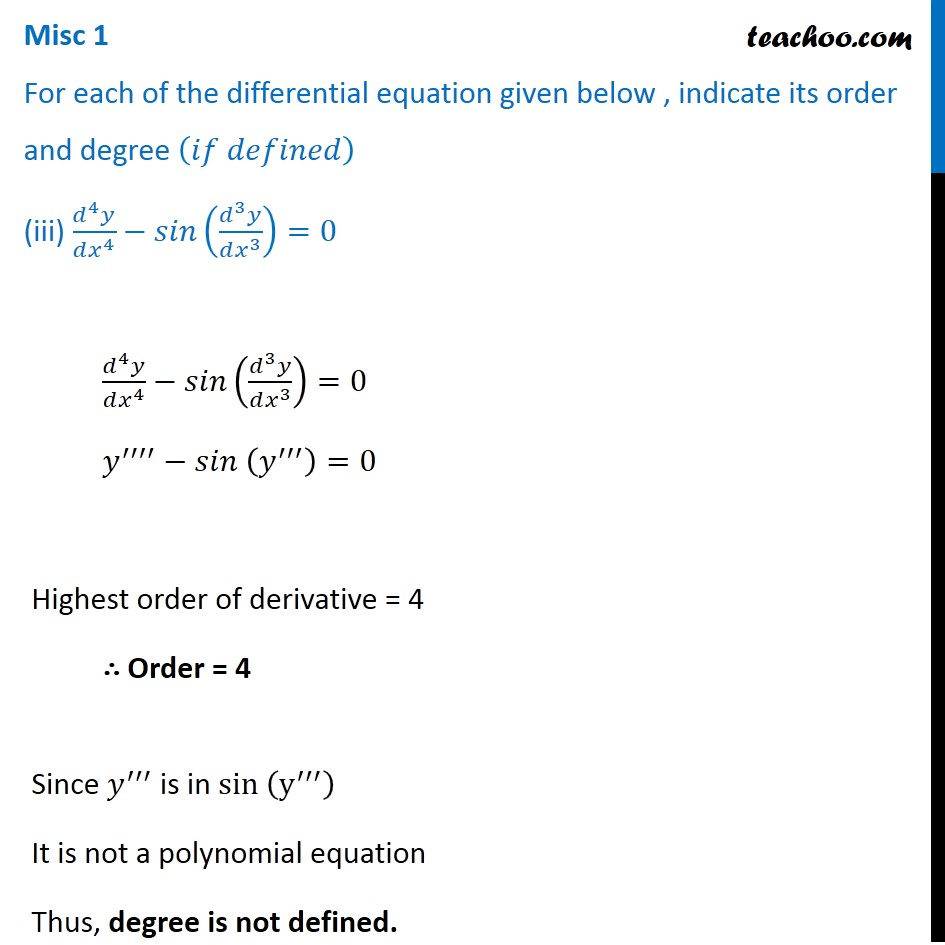Miscellaneous

Chapter 9 Class 12 Differential Equations
Serial order wiseLearn in your speed, with individual attention - Teachoo Maths 1-on-1 Class

### Transcript

Misc 1 For each of the differential equation given below , indicate its order and degree (𝑖𝑓 𝑑𝑒𝑓𝑖𝑛𝑒𝑑) (iii) (𝑑^4 𝑦)/(𝑑𝑥^4 )−𝑠𝑖𝑛((𝑑^3 𝑦)/(𝑑𝑥^3 ))=0 (𝑑^4 𝑦)/(𝑑𝑥^4 )−𝑠𝑖𝑛((𝑑^3 𝑦)/(𝑑𝑥^3 ))=0 𝑦^′′′′−𝑠𝑖𝑛 (𝑦^′′′ )=0 Highest order of derivative = 4 ∴ Order = 4 Since 𝑦^′′′ is in sin (y^′′′ ) It is not a polynomial equation Thus, degree is not defined.